153 11.72 MB 0

## Description of All-In-One Calculator

It's a FREE, complete and easy to use multi calculator & converter.
Designed with simplicity in mind, it helps you solve everyday problems.
From simple or complex calculations, to unit and currency conversions, percentages, proportions, areas, volumes, etc... it does it all. And it does it good!
Passionate development combined with constant feedback we receive from our users resulted in that we think is the best multi calculator on the store.
Featuring over 75 FREE Calculators and Unit Converters packed in with a Simple or Scientific Calculator, it's the only calculator you will need from now on on your device.
Yes, it is free. We think everyone should enjoy this.
If you are a student, teacher, engineer, handyman, contractor or just somebody who struggles with math & conversions, you really should give this one a try.
• Use it for simple or complex calculations
• Convert units or currencies in the same app
• Enjoy easier homework or school assignments
• Learn. See step by step solutions to your calculations
So, on with the features...
• Simple or Scientific layout
• Editable input & cursor
• Copy & Paste support
• Calculation history
• Memory buttons
• Function graphing
• Dec, Hex & Binary
• Floating calculator
• Widget
• Algebra, Geometry, Unit Converters, Finance, Health & Misc
• Currency converter with 160 currencies (available offline)
• Instant results delivered as you type
• Step-by-step solution & formulas
• Smart search for faster navigation
• Create shortcuts on home screen
• Percentage calculator
• Proportion calculator
• Ratio calculator
• Average calculator - arithmetic, geometric and harmonic means
• Equation solver - linear, quadratic and equation system
• Combinations and permutations
• Decimal to fraction
• Fraction simplifier
• Prime number checker
• Greatest common factor & Lowest common multiple calculator
• Random number generator
• Shape calculators for square, rectangle, parallelogram, trapezoid, rhombus, triangle, pentagon, hexagon, circle, circle arc, ellipse
• Body calculators for cube, rect. prism, square pyramid, sq. pyramid frustum, cylinder, cone, conical frustum, sphere, spherical cap, spherical frustum, ellipsoid
• Acceleration converter
• Angle converter
• Length converter
• Energy converter
• Force converter
• Torque converter
• Area converter
• Volume converter
• Volumetric flow converter
• Weight converter
• Temperature converter
• Pressure converter
• Power converter
• Speed converter
• Mileage converter
• Time converter
• Digital storage converter
• Data transfer speed converter
• Numeric base converter
• Roman numerals converter
• Shoe size converter
• Ring size converter
• Cooking converter
• Currency converter with 160 currencies available offline
• Unit price calculator
• Sales tax calculator
• Tip calculator
• Loan calculator
• Simple / Compound interest calculator
• Body mass index - BMI
• Daily calories burn
• Body fat percentage
• Age calculator
• Date calculator
• Time calculator
• Mileage calculator
• Ohm's law calculator - voltage, current, resistance and power
Developed in Transylvania 🇷🇴

0

## Good App Guaranteed

This app passed the security test for virus, malware and other malicious attacks and doesn't contain any threats.•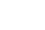•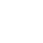•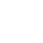•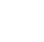•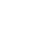2.2.5